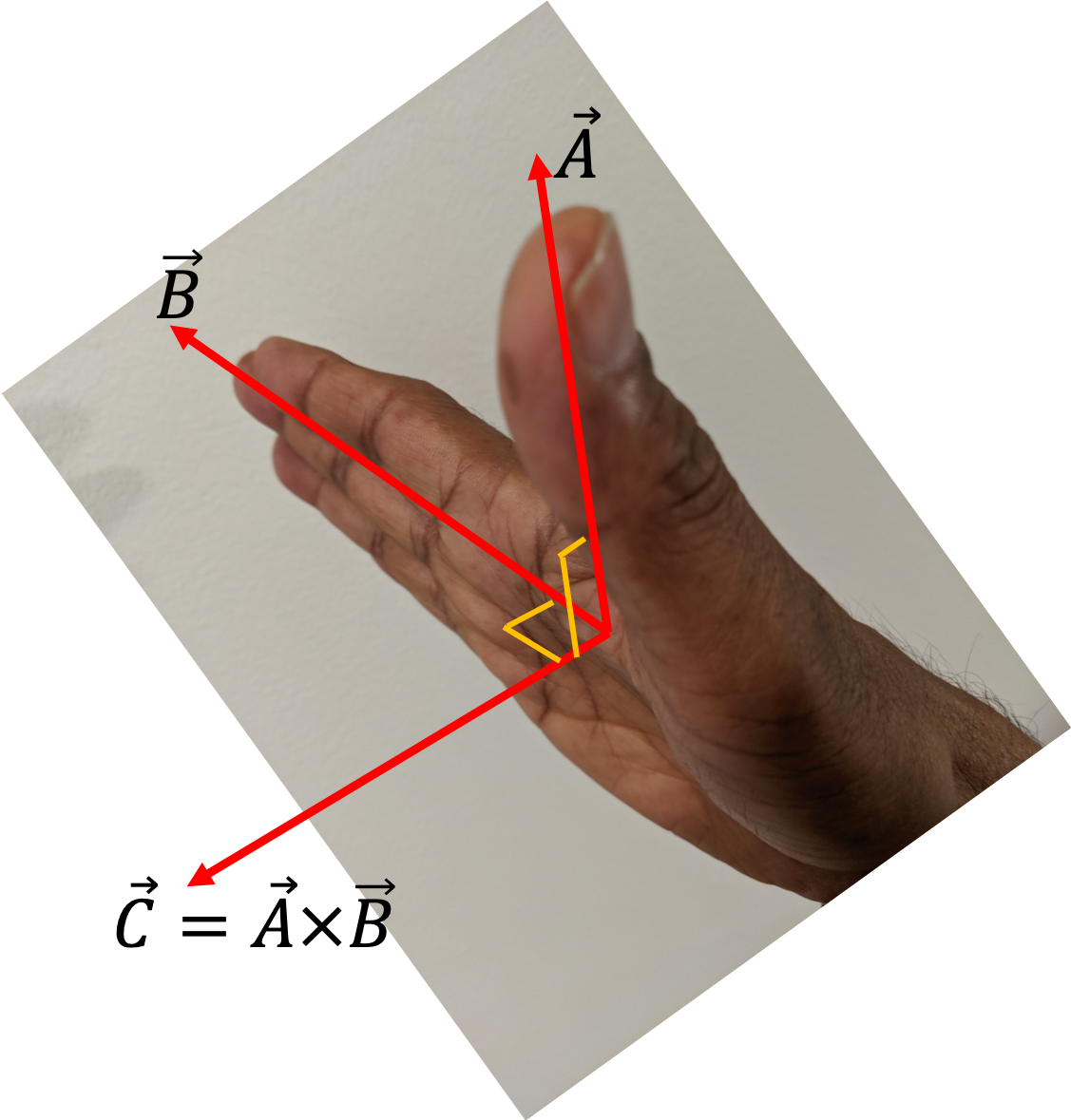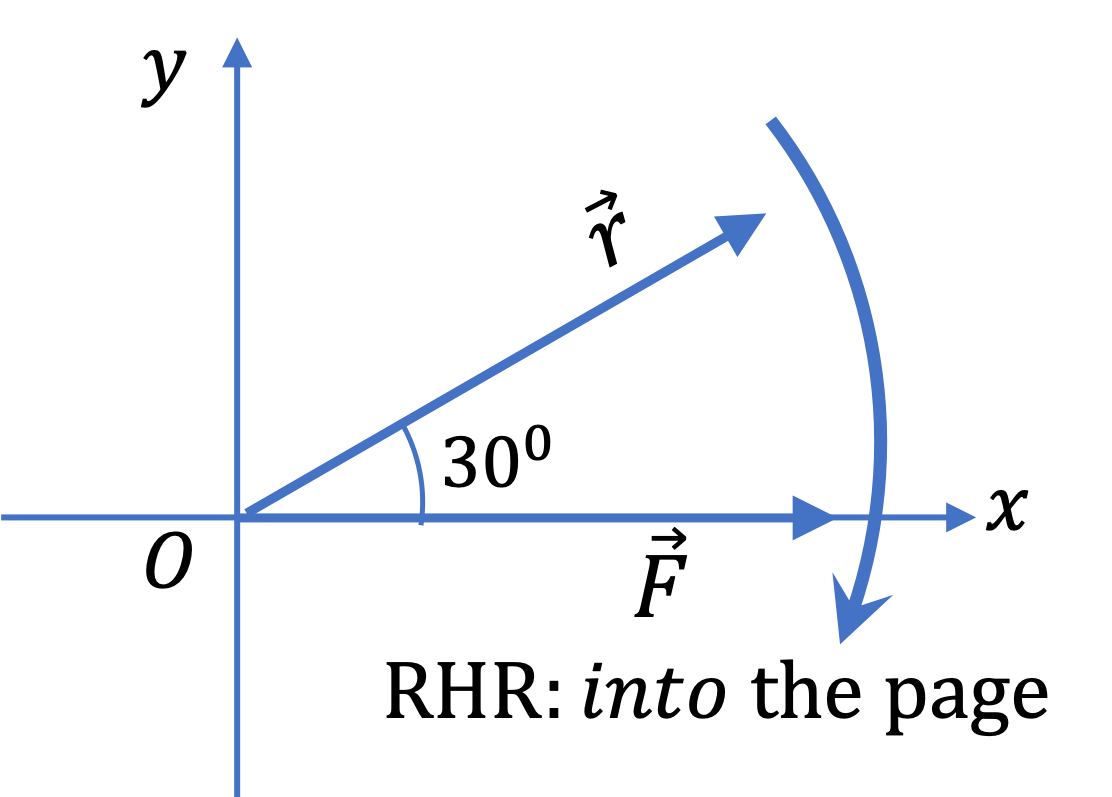## Section3.7Vector Product Between Vectors

In addition to the scalar or dot product we discussed above, there is another type of multiplication between two vectors. We call the second type of multiplication a vector or cross product, since this time, the result is also a vector, not just a simple number as was the case with the dot product. Since, the vector product results in a vector, we need to specify the result either in terms of the component form of the answer or the magnitude and direction form.

Consider two vectors $\vec A$ and $\vec B$ with an angle $\theta$ between them, as illustrated in Figure 3.6.1. The result of a vector or cross product between them, denoted by $\vec A \times \vec B\text{,}$ has the magnitude and direction given by

\begin{align} \amp \text{Magnitude } = A B \sin\theta, \text{ and} \tag{3.7.1}\\ \amp \text{Direction: Use Right Hand Rule described below.}\tag{3.7.2} \end{align}

The Right Hand Rule is illustrated in Figure 3.7.1 You can state the Right Hand Rule as follows: if you point the thumb of your right hand in the direction of vector $\vec A \text{,}$ and point any other finger in the direction of vector $\vec B \text{,}$ then vector $\vec C$ will be perpendicular to your palm and coming out of the palm as shown in the figure.Figure 3.7.1. The Right Hand Rule for vector/cross product. If you point the thumb of your right hand in the direction of vector $\vec A \text{,}$ and point any other finger in the direction of vector $\vec B \text{,}$ then vector $\vec C$ will be perpendicular to your palm and coming out of the palm.

It is often more convenient to write the result of the vector product between vectors $\vec A$ and $\vec B$ in terms of the components of $\vec A$ and $\vec B \text{.}$ Since the result is a vector, we state the components of the result in terms of the components of $\vec A$ and $\vec B \text{.}$

\begin{align} \amp (\vec A \times \vec B)_x = A_y B_z - A_z B_y,\tag{3.7.3}\\ \amp (\vec A \times \vec B)_y = A_z B_x - A_x B_z,\tag{3.7.4}\\ \amp (\vec A \times \vec B)_z = A_x B_y - A_y B_x,\tag{3.7.5} \end{align}

From these components of $\vec A \times \vec B$ or from the way right had rule operates, you can show that the if you change the order of $\vec A$ and $\vec B \text{,}$ then you would get the result pointed in the opposite direction.

\begin{equation} \vec B \times \vec A = - \vec A \times \vec B.\tag{3.7.6} \end{equation}

Another important thing to notice is that the product depends on the angle between the vectors through $\sin\, \theta \text{.}$ Thus, if $\theta = 0$ or $180^{\circ}\text{,}$ the cross prosuct will be zero. That is, the cross product of parallel or anti-parallel vectors is always zero.

Vector $\vec A$ is pointed towards East and vector $\vec B$ is pointed towards North. (a) Which way is the cross product $\vec A \times \vec B$ pointed? (b) Which way is the cross product $\vec B \times \vec A$ pointed?

Hint

Use RHR.

(a) UP, (b) DOWN

Solution

(a) Using right-hand rule, gives the answer "UP" for the direction of $\vec A \times \vec B \text{.}$

(b) Using right-hand rule, gives the answer "DOWN" for the direction of $\vec B\times \vec A \text{.}$

The force on a straight wire of length $L$ carrying a current $I$ placed between the poles of a horse-shoe magnet is given by the formula $I \vec L \times \vec B \text{,}$ where $\vec L$ is a vector of length same as the length of the wire and the direction the same as the direction of current flow, and $\vec B$ is the magnetic field vector.

In a physics lab, $L = 0.20 \text{ meter}\text{,}$ $I = 10.0 \text{ Amperes}\text{,}$ and $B = 1.5 \text{ Tesla} \text{.}$ The direction of $\vec B$ is up and the direction of current flow is North. (a) What is the angle between $\vec L$ and $\vec B \text{?}$ (b) What are the magnitude and direction of the force on the wire?

Hint

(a) $\theta=90^\circ\text{,}$ (b) Use definition.

(a) $90^{\circ}\text{,}$ (b) $3\text{ N}$ due East.

Solution

(a) The angle between Up and North directions is $90^{\circ}\text{.}$

(b)

\begin{align*} \amp\text{Magnitude = } ILB\sin\theta=10\times 0.2\times 1.5\times \sin\, 90^{\circ} = 3\,\text{A.m.T} \\ \amp\text{Direction; Using right hand rule we get due East. } \end{align*}

The unit of force came out to be $\text{A.m.T} \text{,}$ which actually turns out to be same as $\text{N} \text{.}$

A particle located at $(x, y, z) = (3, -2, 5) \text{,}$ where distances are in the unit $\text{m} \text{,}$ is subject to a force whose components are $(F_x, F_y, F_z) =$$(-10, 30, -20 )$ in units of $\text{N}\text{.}$ Find the components of torque on the particle given by $\vec r \times \vec F\text{.}$

Hint

Use components of $\vec A \times \vec B\text{.}$

$-110\text{ N.m }\hat i + 10\text{ N.m }\hat j + 70\text{ N.m }\hat k.$

Solution

You may recall that $x, \, y,\, z$ are the components of the position vector $\vec r \text{.}$ Therefore to get the components of $\vec r \times \vec F$ we just use the formulas given.

\begin{align*} (\vec r \times \vec F)_x \amp = y F_z - z F_y \\ \amp = (-2)\times(-20) - 5\times 30 = -110 \end{align*}
\begin{align*} (\vec r \times \vec F)_y \amp = z F_x - x F_z \\ \amp = 5\times(-10) - 3\times (-20) = 10 \end{align*}
\begin{align*} (\vec r \times \vec F)_z \amp = x F_y - y F_x \\ \amp = 3\times 30 - (-2)\times (-10) = 70 \end{align*}

Therefore,

\begin{equation*} \vec \tau = -110\text{ N.m }\hat i + 10\text{ N.m }\hat j + 70\text{ N.m }\hat k. \end{equation*}

Consider a force vector $\vec F$ that has a magnitude $25.0\text{ N}$ in the direction of East and a displacement vector $\vec r$ that has a magnitude $4.0\text{ m}$ in the direction of $30^{\circ}$ North of East. For stating directions in space, let $x$ axis be pointed towards East, $y$ axis be pointed towards North, and $z$ axis be pointed Up. Find (a) $\vec r \cdot \vec F \text{,}$ and (b) $\vec r \times \vec F \text{.}$

Hint

Use formulas in terms of magnitudes and angle.

(a) $86.6\text{ N.m} \text{,}$ (b) ($50.0\text{ N.m} \text{,}$ in the negative $z$ axis direction).

Solution

Since magnitudes of the two vectors and the angle between them are given, we can just use the defining formula for the dot product to get the value of the dot product and magnitude of the cross product. To find the direction of the cross product, we use the right hand rule given in the definition.

(a) The dot product:

\begin{align*} \vec r \cdot \vec F \amp = r\, F\, \cos\, \theta \\ \amp = 4.0\text{ m}\times 25.0\text{ N}\times\cos\, 30^{\circ} = 86.6\text{ N.m}. \end{align*}

(b) The cross product $\vec r \times \vec F \text{:}$

\begin{align*} \text{Magnitude } \amp = r\, F\, \sin\, \theta \\ \amp = 4.0\text{ m}\times 25.0\text{ N}\times\sin\, 30^{\circ} = 50.0\text{ N.m}. \end{align*}

We obtain the direction of $\vec r \times \vec F$ vector by using the right hand rule. One way to do that is shown in this figure. I sweep my right hand from $\vec r$ towards $\vec F \text{,}$ then the thumb points down into the page, which is the negative $z$ axis direction. Another way is to point thumb in the direction of $\vec r$ and any of the other fingers towards $\vec F \text{,}$ then you would find that your palm points down.Consider a force vector $\vec F$ that has a magnitude $25.0\text{ N}$ in the direction of East and a displacement vector $\vec r$ that has a magnitude $4.0\text{ m}$ in the direction of $30^{\circ}$ South of East. For stating directions in space, let $x$ axis be pointed towards East, $y$ axis be pointed towards North, and $z$ axis be pointed Up. Find (a) $\vec r \cdot \vec F \text{,}$ and (b) $\vec r \times \vec F \text{.}$

Hint

Use formulas.

(a) $86.6\text{ N.m} \text{,}$ (b) ($50.0\text{ N.m} \text{,}$ in the positive $z$ axis direction).

Solution

See Checkpoint 3.7.5. The only thing new is the result of the right hand rule.

Consider a force vector $\vec F$ that has a magnitude $25.0\text{ N}$ in the direction of East and a displacement vector $\vec r$ that has a magnitude $4.0\text{ m}$ in the direction of West. For stating directions in space, let $x$ axis be pointed towards East, $y$ axis be pointed towards North, and $z$ axis be pointed Up. Find (a) $\vec r \cdot \vec F \text{,}$ and (b) $\vec r \times \vec F \text{.}$

Hint

Use formulas.

Ans: (a) $-100.0\text{ N.m} \text{,}$ (b) ($0 \text{,}$ indeterminate direction).

Solution

Here the angle between the two vectors are $180^{\circ} \text{.}$ Therefore, we have

\begin{equation*} \cos\,\theta = -1,\ \ \sin\, \theta = 0. \end{equation*}

Therefore, the dot product will give $\vec r \cdot \vec F = -100.0\text{ N.m}\text{,}$ and the magnitude of the cross product will be $100.0 \times 0 = 0\text{.}$ Since the magnitude of the cross product is zero, it is zero vector, and hence its direction is indeterminate.

Consider a force vector $\vec F$ that has a magnitude $25.0\text{ N}$ in the direction of East and a displacement vector $\vec r$ that has a magnitude $4.0\text{ m}$ in the direction of North. For stating directions in space, let $x$ axis be pointed towards East, $y$ axis be pointed towards North, and $z$ axis be pointed Up. Find (a) $\vec r \cdot \vec F \text{,}$ and (b) $\vec r \times \vec F \text{.}$

Hint

Use definitions.

(a) $0 \text{,}$ (b) ($100.0\text{ N.m} \text{,}$ in the negative $z$ axis direction).
Here the angle between the two vectors are $90^{\circ} \text{.}$ Therefore, we have
Therefore, the dot product will give $\vec r \cdot \vec F = 0\text{,}$ and the magnitude of the cross product will be $100.0 \text{ N.m}\text{.}$ Using the right-hand rule, you can deduce the direction to be into-the-page direcion, which is the direction of the negative $z$ axis.